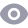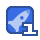### c++小程序Fibonnaci0收藏

c++小程序Fibonnaci //非递归编写fibonacci数列f(n)={1，n=1;1,n=2;f(n-1)+f(n-2)} int f(int n){ if(n==1||n==2)return 1; for(int a=1,b=1,c=0,j=2;j<n;){ c=a+b; a=b; b=c; } return c; } //递归方式 int f1(int n){ if(n==1||n==2)return 1; return f1(n-1)+f1(n-2); }

...展开详情

• 5
资源
• 0
粉丝
•等级

最新推荐